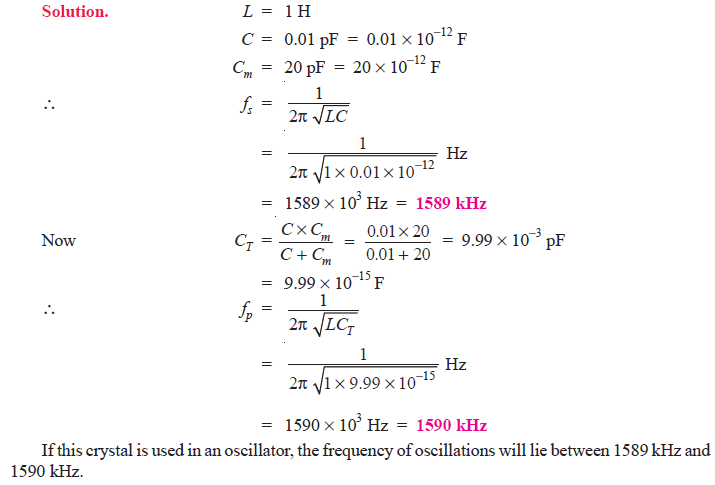Q1. The tuned collector oscillator circuit used in the local oscillator of a radio receiver makes use of an LC tuned circuit with L1 = 58.6 μH and C1 = 300 pF. Calculate the frequency of oscillations.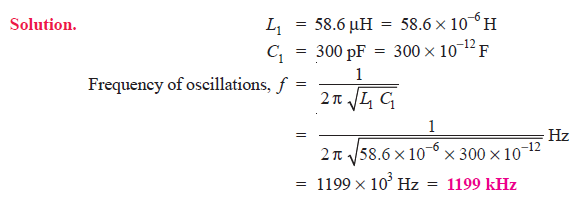Q2. Find the capacitance of the capacitor required to build an LC oscillator that uses an inductance of L1 = 1 mH to produce a sine wave of frequency 1 GHz (1 GHz = 1 × 1012 Hz).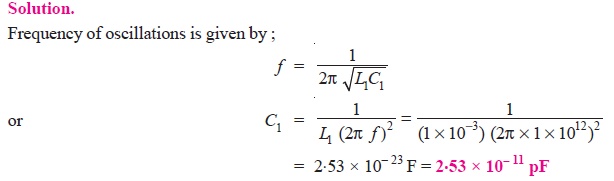Q3. Determine the (i) operating frequency and (ii) feedback fraction for Colpitt’s oscillator shown in Fig. 1.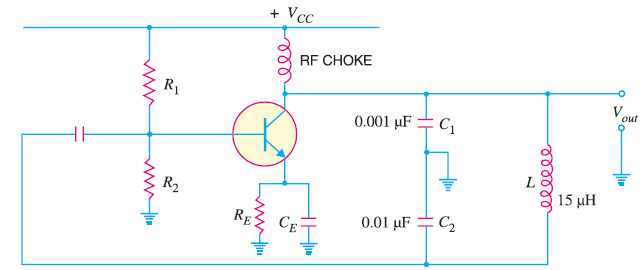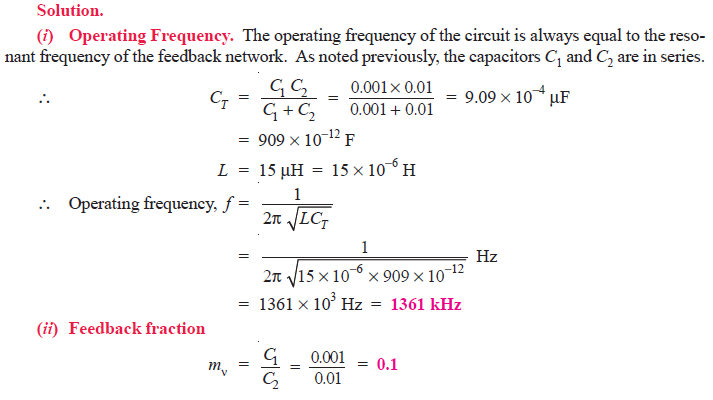Q4. A 1 mH inductor is available. Choose the capacitor values in a Colpitts oscillator so that f = 1 MHz and mv = 0.25.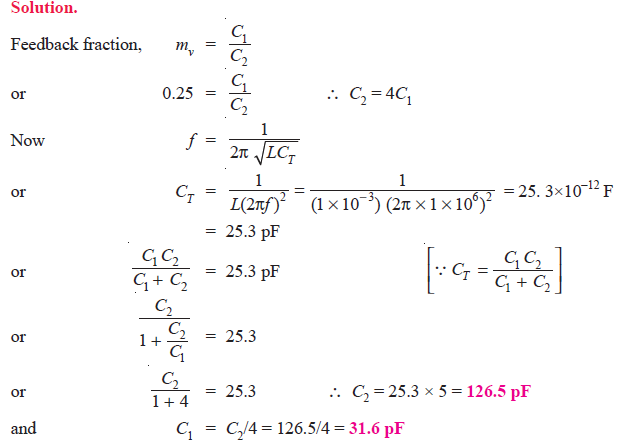Q5. Calculate the (i) operating frequency and (ii) feedback fraction for Hartley oscillator shown in Fig. 2. The mutual inductance between the coils, M = 20 μH.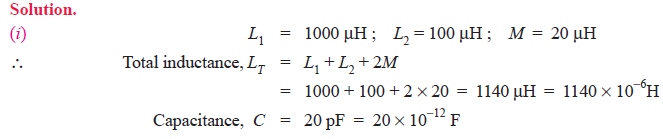Q6. A 1 pF capacitor is available. Choose the inductor values in a Hartley oscillator so that f = 1 MHz and mv = 0.2.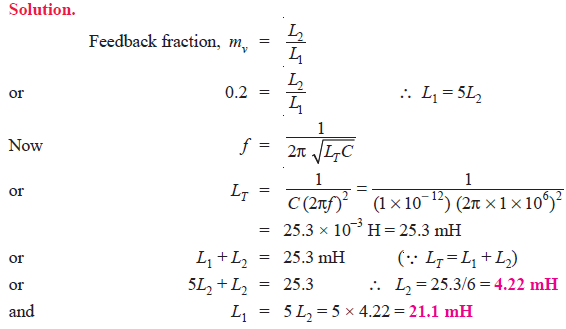Q7. In the phase shift oscillator shown in Fig. 3, R1 = R2 = R3 = 1MΩ and C1 = C2 = C3 = 68 pF. At what frequency does the circuit oscillate ?Fig. 3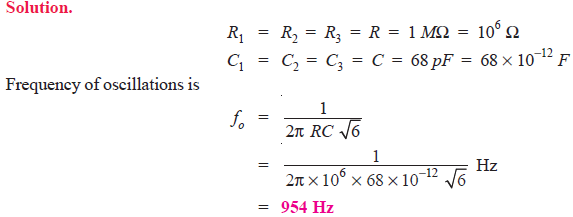Q8. A phase shift oscillator uses 5 pF capacitors. Find the value of R to produce a frequency of 800 kHz.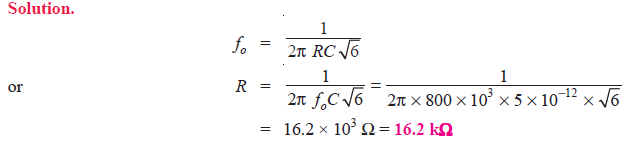Q9. In the Wien bridge oscillator shown in Fig. 4 , R1 = R2 = 220 kΩ and C1 = C2 = 250 pF. Determine the frequency of oscillations.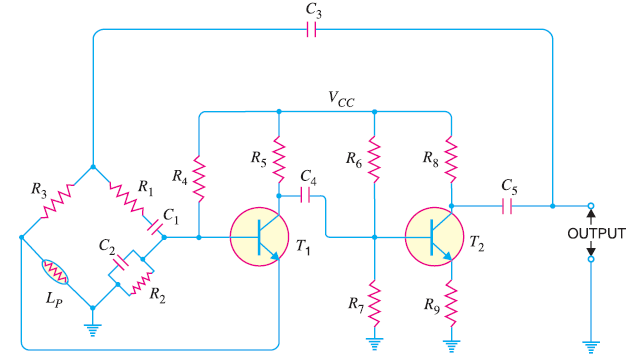Fig. 4Q10.The ac equivalent circuit of a crystal has these values: L = 1H, C = 0.01 pF, R = 1000 Ω and Cm = 20 pF. Calculate fs and fp of the crystal.